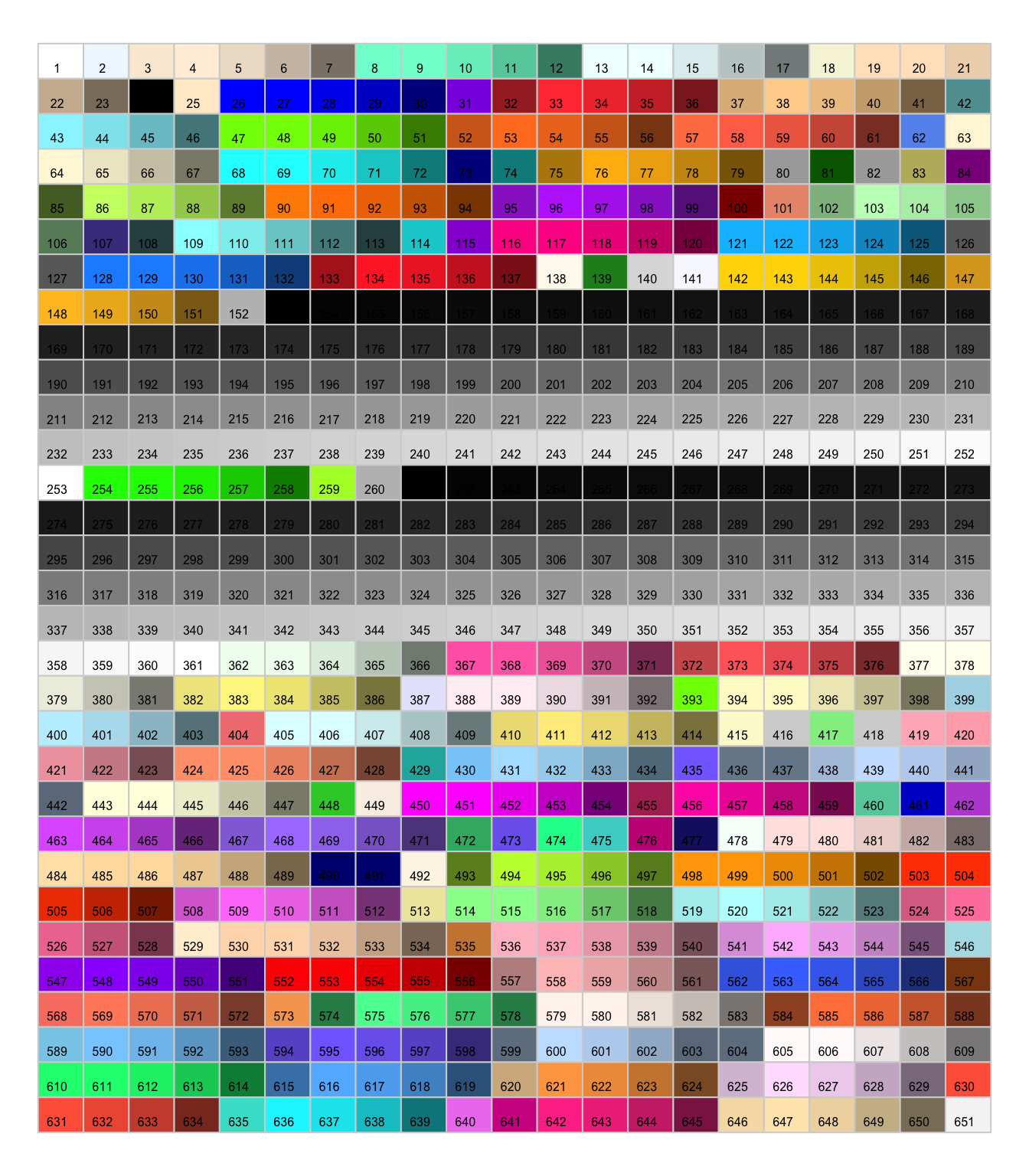# An overview of color numbers in R

This post displays more than 200 colors that can be called by a number in `R`. In case you’re struggling to find the one that suits you the best.

In `R` you can call colors by their numbers. This picture shows corespondances between numbers and colors.

For example, if you call `col=colors()`, You will get a kind of yellow.``````par(mar=c(0,0,0,0))
plot(0, 0, type = "n", xlim = c(0, 1), ylim = c(0, 1), axes = FALSE, xlab = "", ylab = "")

# Parameters
line <- 31
col <- 21

# Rectangles
rect( rep((0:(col - 1)/col),line) ,  sort(rep((0:(line - 1)/line),col),decreasing=T) , rep((1:col/col),line) , sort(rep((1:line/line),col),decreasing=T),
border = "light gray" , col=colors()[seq(1,651)])

# Text
text( rep((0:(col - 1)/col),line)+0.02 ,  sort(rep((0:(line - 1)/line),col),decreasing=T)+0.01 , seq(1,651)  , cex=0.5)``````

Related chart types

## Contact

This document is a work by Yan Holtz. Any feedback is highly encouraged. You can fill an issue on Github, drop me a message on Twitter, or send an email pasting yan.holtz.data with gmail.com.